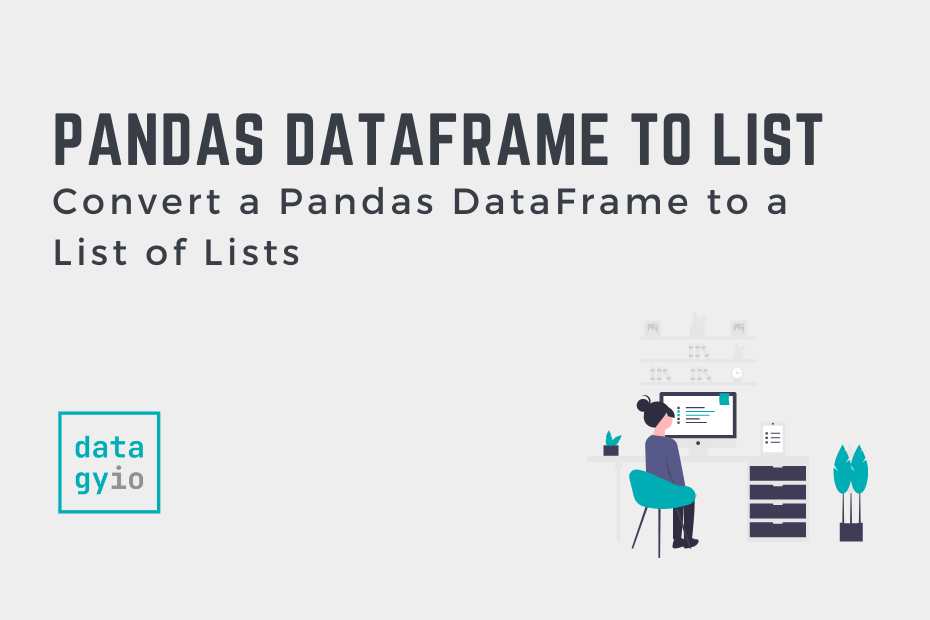# Convert a Pandas DataFrame to a ListIn this post, you’ll learn how to convert a Pandas DataFrame to a list, including a list of lists, a list of tuples, and a list of dictionaries. Being able to convert a Pandas DataFrame to different formats allows you to work with different libraries that may not accept Pandas DataFrames. Pandas provides you with a large number of options for converting your data to other formats.

By the end of this tutorial, you’ll have learned:

• How to convert a Pandas DataFrame to a list of lists
• How to convert a Pandas DataFrame column (or Series) to a list
• How to convert a Pandas DataFrame to a list of tuples
• How to convert a Pandas DataFrame to a list of dictionaries

Below, you’ll find code to load a sample Pandas DataFrame that you can use to follow along with. If you have your own dataset, feel free to use that. However, your results will of course vary.

``````# Loading a Sample Pandas DataFrame
import pandas as pd

df = pd.DataFrame({
'Name': ['Nik', 'Kate', 'Evan', 'Kyra'],
'Age': [33, 34, 43, 44],
'Score': [90, 95, 93, 92]
})

print(df)

# Returns:
#    Name  Age  Score
# 0   Nik   33     90
# 1  Kate   34     95
# 2  Evan   43     93
# 3  Kyra   44     92``````

You can see from the code above that you have loaded a Pandas DataFrame with three columns and four records. The dataset is deliberately simple, in order for you to see the entire process.

## Convert a Pandas DataFrame to a List of Lists

In this section, you’ll learn how to convert a DataFrame to a list of lists. Pandas DataFrames have an attribute, `.values`, which contains all of the values in DataFrame represented as a NumPy array. NumPy arrays have a method available, `.tolist()`, which converts an array to a list.

Let’s see what this looks like:

``````# Convert a Pandas DataFrame to a List of Lists
import pandas as pd

df = pd.DataFrame({
'Name': ['Nik', 'Kate', 'Evan', 'Kyra'],
'Age': [33, 34, 43, 44],
'Score': [90, 95, 93, 92]
})

values = df.values.tolist()
print(values)

# Returns:
# [['Nik', 33, 90], ['Kate', 34, 95], ['Evan', 43, 93], ['Kyra', 44, 92]]``````

In the code block above, we accessed the values of the DataFrame and then applied the `.tolist()` method. This returns a list of lists, where each sublist is a record in the DataFrame.

In the following section, you’ll learn how to convert a Pandas Series to a list.

## Convert a Pandas Series (Column) to a List

In order to convert a Pandas Series to a list, you can either apply the `.tolist()` to a Pandas Series or pass the Series into the `list()` function. These methods accomplish the same thing! Let’s take a look at how we can use either of these methods to convert the `'Age'` column to a list:

``````# Convert a Pandas Series to a List
import pandas as pd

df = pd.DataFrame({
'Name': ['Nik', 'Kate', 'Evan', 'Kyra'],
'Age': [33, 34, 43, 44],
'Score': [90, 95, 93, 92]
})

list1 = df['Age'].values.tolist()
list2 = list(df['Age'])

print('list1 contains: ', list1)
print('list2 contains: ', list2)

# Returns:
# list1 contains:  [33, 34, 43, 44]
# list2 contains:  [33, 34, 43, 44]``````

We can see that both methods explained above return the same list of values. The first method, using `df['Age'].value.tolist()` can be more intentional and descriptive of what you’re hoping to accomplish.

In the following section, you’ll learn how to convert a DataFrame to a list of tuples.

## Convert a Pandas DataFrame to a List of Tuples

In order to convert a Pandas DataFrame into a list of tuples, you can use the `.to_records()` method and chain it with the `.tolist()` method. The `.to_records()` method converts the DataFrames records into tuple-like containers. When the `.tolist()` method is then applied, the object is converted to a Python list.

Let’s see what this process looks like:

``````# Convert a Pandas DataFrame to a List of Tuples
import pandas as pd

df = pd.DataFrame({
'Name': ['Nik', 'Kate', 'Evan', 'Kyra'],
'Age': [33, 34, 43, 44],
'Score': [90, 95, 93, 92]
})

list_of_tuples = df.to_records().tolist()
print(list_of_tuples)

# Returns:
# [(0, 'Nik', 33, 90), (1, 'Kate', 34, 95), (2, 'Evan', 43, 93), (3, 'Kyra', 44, 92)]``````

In the next section, you’ll learn how to convert a DataFrame to a list of dictionaries.

## Convert a Pandas DataFrame to a List of Dictionaries

In order to convert a Pandas DataFrame to a list of dictionaries, you can use the `.to_dict()` method. The method provides a number of different ways in which you can convert your Pandas DataFrame.

By passing in `'records'` into the method, the DataFrame is converted into a list of dictionaries. This can be helpful when moving the DataFrame into other languages, given how closely the format matches a JSON-style format.

Let’s see how we can accomplish this:

``````# Convert a Pandas DataFrame into a List of Dictionaries
import pandas as pd

df = pd.DataFrame({
'Name': ['Nik', 'Kate', 'Evan', 'Kyra'],
'Age': [33, 34, 43, 44],
'Score': [90, 95, 93, 92]
})

list_of_dicts = df.to_dict('records')
print(list_of_dicts)

# Returns:
# [{'Name': 'Nik', 'Age': 33, 'Score': 90}, {'Name': 'Kate', 'Age': 34, 'Score': 95}, {'Name': 'Evan', 'Age': 43, 'Score': 93}, {'Name': 'Kyra', 'Age': 44, 'Score': 92}]``````

## Conclusion

In this post, you learned how to convert a Pandas DataFrame into a list. This process can be helpful when moving a dataset between libraries (or even languages). You first learned how to convert a DataFrame to a list, then how to convert a Series to a list. You also learned how to convert a DataFrame to a list of tuples and a list of dictionaries.